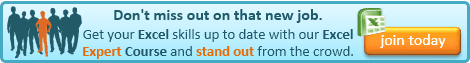## Tuesday, 7 January 2014

### Excel Tip: The structure of every Excel function

As this is my first post of 2014, I must start by wishing all of my readers a very Happy New Year, and thank you all for making 2013 the most successful yet for Not Just Numbers.

I have explained hundreds of Excel functions on this blog, and, in one post, covered how to access every function in Excel, but it occurred to me that I have never covered the universal way that every Excel function works, so I thought that would be a useful way to start the New Year.

Every function in Excel follows exactly the same format, and when you understand that, it makes it much easier to pick up new functions.

The basic format of an Excel function is the name of the function, followed by its arguments in brackets and separated by commas:

=FUNCTIONNAME(argument1,argument2...)

I have included the equals sign (=) in front of the format above but this is not strictly part of the individual function. The equals sign (as the first character in a cell) tells Excel that the contents of that cell are to be evaluated. So, if the function was entered in a cell on its own, it would need the equals sign - however if the function was used within another function or calculation, you would not repeat the equals sign.

The arguments of the function are the values that it needs to know in order to calculate. The number of arguments will differ depending on the function. Some functions also have optional arguments (in the Excel help files, these are shown in square brackets.

Let's take the IF function as an example. In programming, an IF statement would normally follow the following syntax:

IF logical_test THEN value_if_true ELSE value_if_false

However, in Excel, it must follow the Excel format for all functions as described above, so the three arguments are separated by commas in brackets after the function name, i.e.

IF(logical_test,value_if_true,value_if_false)

Its format, according to the Excel help files, is:

IF(logical_test, [value_if_true], [value_if_false])

As we can see, only the first argument is required (logical_test) the other two being optional (value_if_true and value_if_false).

What is acceptable for each argument will depend on the function in question, but in this case, logical_test is an expression that can be evaluated as true or false, whereas value_if_true and value_if_false can be any value (numbers or text) or any calculation resulting in a value.

So some acceptable IF statements could be:

=IF(A1=10)   returns TRUE if A1=10, or FALSE otherwise
=IF(A1=10,"Yes","No")   returns Yes if A1=10, or No otherwise

or even,

=IF(A1=10,"Yes",IF(B1=5,"Yes","No"))   returns Yes if A1=10, but if A1 doesn't equal 10,  it returns Yes if B1 is 5 but No otherwise

Yes, functions can be used as arguments for other functions (including other instances of the same function - as in this example).

The last example is a Nested IF statement. Don't worry if you haven't followed fully how the IF statement works, as this was not the point of this post. You can read more about the IF statement here.

The important thing is that you understand the structure of Excel functions in general.If you enjoyed this post, go to the top of the blog, where you can subscribe for regular updates and get two freebies "The 5 Excel features that you NEED to know" and "30 Chants for Better Charts".

1.1.